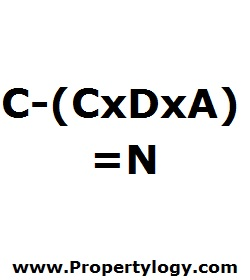Formula - Net Current Property Value | Propertylogy
Don't Miss

# Formula – Net Current Property Value

By on June 18, 2018C – (C x D x A) = N

C – Cost of improvements

D – Rate of depreciation

A – Effective age

N – Net current property value

Following up with the formula for calculating the property value based on Cost Approach, a better estimated property value can be determined by factoring in depreciation based on the effective age of the property in question.

For example, if cost of real estate improvements is \$100,000, with depreciation of 2.5% and effective age of 6 years, the net current property value will be:

\$100,000 – (\$100,000 x 2.5% x 6) = \$85,000

For an appraiser, the key concern is determining the effective age after working out appraisal depreciation.

How accurate effective age will then depend on the judgment and experience of the appraiser.

Send this to a friend